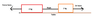# Two blocks connected with a fragile rope

• I
• ChessEnthusiast

#### ChessEnthusiast

Let's say that we have two blocks - 2 kg and 1 kg.
They are connected with a rope that can stand the maximal tension of 20 N.

Does the maximal force we can apply to the block so that the rope does not break apart depend on the choice of the block?

A diagram could help here. Does this involve a pulley? It's impossible to suggest and answer at the moment.

•Dale and russ_watters
There is no pulley. We simply pull one of the block so that it increases the tension.

There is no pulley. We simply pull one of the block so that it increases the tension.
A diagram would still help. Horizontal or vertical? Fast or slow application of force? Friction? Stationary or accelerating?

The answer is probably yes, but I can think of scenarios where it could be no.

•sophiecentaur
Are the blocks floating in space or is there some friction involved. Without a diagram neither of us can help you.

I have drawn a diagram.

#### Attachments

•forces2.PNG
3.2 KB · Views: 413
If the force applied is very slow then it makes no difference. If the force is applied in a very quick jerk then it makes a big difference.

The tension in the rope will depend on which side you apply the force. Even for the stationary case (or very slow application of force). Actually the fast application of force is the one that may break the rope in both cases.

I have drawn a diagram.
That's more useful. Thanks
Yes, it's all to do with F=ma.
I am assuming that the applied force is ramped up slowly enough to avoid any stretching of the rope and no jerking.
For a start, the block at the end that's pulled will make no difference to the tension in the rope; we are just concerned with the 'other' block.
If the rope will stand 20N then the maximum acceleration it can give to the 10kg block will be 20/10 = 2m/s2. It can only give the 20kg block 20/20 = 1m/s2
To accelerate both blocks, the Force needed will be total mass X acceleration so the force when pulling the 20kg block directly can be as high as 30X2 = 60N and for pulling the 10kg block directly the force can be no more than 30N.
Any other practical details would need to be added in and the calculation would be much more complicated.

•ChessEnthusiast